## teaching and educationJOURNAL OFAPPLIEDCRYSTALLOGRAPHY
ISSN: 1600-5767
Volume 50| Part 5| October 2017| Pages 1541-1544

## Direction indices for crystal lattices

aUniversité de Lorraine, CNRS, CRM2, Nancy, France
*Correspondence e-mail: massimo.nespolo@univ-lorraine.fr

(Received 30 June 2017; accepted 17 July 2017; online 9 August 2017)

Direction indices [uvw] of rational directions in crystal lattices are commonly restricted to integer numbers. This restriction is correct only when primitive unit cells are used. In the case of centred cells, however, direction indices may take fractional values too, because the first lattice node after the origin along a direction can have fractional coordinates in a centred basis. This evidence is very often overlooked and an undue simplification of direction indices to integer values is usually adopted. Although such a simplification does not affect the identification of the direction, it is potentially a source of confusion and mistakes in crystallographic calculations. A parallel is made with the incorrect restriction of Miller indices to relatively prime integers in centred cells.

### 1. Introduction

A misunderstanding frequently showing up in the crystallographic textbooks states that Miller indices of lattice planes are always relatively prime. As we have pointed out (Nespolo, 2015a), this is true only when a primitive unit cell is adopted, whereas in the case of multiple unit cells this condition no longer applies and is replaced by characteristic relations between Miller indices that correspond to the integral reflection conditions.

Miller indices and direction indices are contravariant, which means that under a certain transformation, like a change of basis, they are transformed by matrices that are the inverse of one another. We can therefore expect that the different restrictions applying to Miller indices as a function of the type of unit cell adopted find a counterpart in similar restrictions on the direction indices. This evidence seems generally overlooked and may result in serious mistakes in crystallographic calculations.

Whereas a clear, although not always correct, definition of Miller indices appears in every crystallographic textbook, a similarly precise definition of direction indices is often absent, even in International Tables for Crystallography, probably because the concept is considered trivial. As we are going to show, this is actually not the case.

Mauguin (1924) defined rational directions through the equation (in the original notation)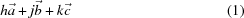(p. 11) and used the symbol [hjk] (today [uvw]) to represent the direction corresponding to the equation above. He explicitly stated that h, j and k are integers. However, the unit cells adopted by Mauguin are all primitive, which justifies the restriction.

In a similar way, Buerger, in his book Elementary Crystallography (Buerger, 1956), defined the direction indices [uvw] by taking a line passing through the origin as a linear combination of three non-coplanar vectors: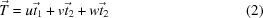(p. 18). Like Mauguin before him, Buerger defined the three integers uvw as indices of the line defined by the vector equation above. He stated that uvw must not have common factors because it is customary to designate a line by the use of the three smallest permissible indices' (our emphasis). The geometric meaning of this statement is that as indices [uvw] we have to take the coordinates uvw of the first lattice node along that direction after the origin. These coordinates, however, are always integers only if the unit cell is primitive, whereas this is no longer true in the case of centred cells. For example, the first lattice node along the positive diagonal of a C-centred unit cell is,, 0 so that, rigorously speaking, the indices of that direction should be [0], or, and not , as would be the case for a primitive unit cell. In fact, to indicate that direction as  implicitly means that the first lattice node along it has coordinates 1, 1, 0, whereas these are the coordinates of the second lattice node. Quite interestingly, the restriction to integer values is not present in Buerger's book X-ray Crystallography (Buerger, 1942, p. 7), where the equation of the rational lattice direction' OP from the origin O to a point P is written vectorially already in modern notation as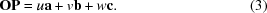The absence of restriction to integer values is consistent with the analysis of the information that can be obtained from an X-ray diffraction pattern which follows, where integral systematic absences suggest the use of centred cells.

It is usually implicitly understood that the fractional factor common to direction indices (in our example above) is omitted for the sake of simplicity. This does not normally result in any problem as long as the direction is considered as stand-alone; however, when a change of basis is applied, as is very often necessary in theoretical and experimental work (in the study of phase transitions, twinning, derivative structures, group–subgroup relations and so on), this apparently innocent simplification may lead to some serious confusion and potentially to wrong results.

### 2. Effects of change of basis on the representation of lattice elements

Let (abc) be the basis vectors used to describe a certain crystal structure and let us suppose that for a certain purpose we want to describe it in a different basis (abc′) with the origin in common.1 Let P be the matrix relating the two bases; the ith column of the P matrix gives the components of the ith vector of the new basis in terms of the old basis: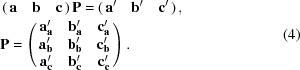The corresponding transformation of Miller indices and direction indices is obtained as follows: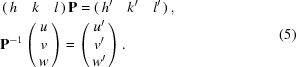As an example, let us suppose we want to describe in a primitive basis a crystal structure whose conventional unit cell is I centred. The transformation matrix is the following (Wondratschek et al., 2016):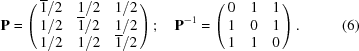Let us consider the family of lattice planes with Miller indices h = k = l and the direction with indices u = v = w. If we assume, as often incorrectly done, that h = 1 and u = 1, i.e. that the Miller and direction indices are (111) and , in the primitive unit cell they transform to () and , respectively. This result is inconsistent because (1) the Miller indices become fractional, contrary to the definition, and (2) the lattice node with coordinates 2, 2, 2 is the second one after the origin along the direction u = v = w, and these coordinates do have a common factor, contrary to the definition. The correct indices in the primitive unit cell are, quite obviously, (111) and , respectively. By applying the inverse transformation, we obtain the correct indices in the I-centred unit cell, i.e. (222) and [], respectively.

One could object that Miller indices and direction indices can be systematically simplified to avoid having common factors or fractional values. Such a simplification is actually a source of potential problems and errors in some data treatment. In fact, there are two points to consider here:

(1) Simplification of Miller indices leads to inconsistencies, namely the density of lattice planes as obtained from the Miller indices becomes incorrect and the very straightforward relation between Miller indices and integral reflection conditions is lost [this has been discussed in detail elsewhere (Nespolo, 2015); interested readers are referred to that publication].

(2) Simplification of direction indices leads to overestimating the periodicity along that direction and obtaining wrong results about the supercell built on it, as we are going to show in the next section.

### 3. An example of errors induced by incorrect restrictions on direction indices

In a number of situations we need to find and characterize a sublattice (based on a supercell of the original lattice): phase transitions with a group–subgroup relation, derivative structures, twinning [see the general approach described by Müller (2013)]. The correct indexing of lattice planes and directions is mandatory to avoid oversights and mistakes. We illustrate the potential problems through an example worked out in detail.

Sulfur crystallizes in Fddd with cell parameters a = 10.4646, b = 12.8660, c = 24.4860 Å (Rettig & Trotter, 1987). Three twin laws are known in the literature; below we analyse twinning by reflection on (101). The unit cell of the twin lattice is built on this plane and the lattice direction quasi-perpendicular to it, which, according to the procedure described by Nespolo (2015b), would be  if the simplification described in the previous section is adopted. In fact, the direction perpendicular to (hkl) is [hkl]*, which in direct space corresponds to an irrational direction obtained as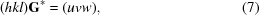where G* is the metric tensor in reciprocal space. By inserting the cell parameters and the Miller indices from the literature, we obtain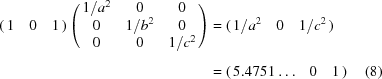after normalization with respect to the smallest nonzero value. The closest rational directions corresponding to a reasonable twin index are  (twin index 3, obliquity 1.94°) and  (twin index 7, obliquity 1.84°) (calculation performed with the software geminography: Nespolo & Ferraris, 2006), of which the former is clearly the best description of the twin.

Actually, neither (101) nor  is the correct description of the lattice elements defining the unit cell of the twin lattice. In fact, the first lattice plane of the family (h0h) in an F-centred cell has intercepts on the a and c axes at, not 1, so that the correct Miller indices are (202). Furthermore, the direction that passes through the origin and the lattice node 5, 0, 1 actually passes also through the lattice node, 0,so that the direction indices are. If we blindly compute the cell parameters of the twin lattice of the basis of the simplified Miller and direction indices, we get a wrong result and a gross overestimation of the twin index. In fact, the transformation matrix in equation (1)contains the two shortest directions in the twin plane and the direction quasi-perpendicular to it, namely ,  and [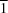01], arranged so as to obtain a positive determinant, i.e. 6 in this case. Knowing that the unit cell of the individual crystal is F centred, that the unit cell based on (101) and  is B centred (see Fig. 1and discussion below), and that the twin index is n = det(P)fi/fT, where f is the unit-cell centring factor of the corresponding cell (Nespolo, 2016), one would be tempted to conclude that the twin index is n = 6 × 4/2 = 12. The mistake becomes evident on inspection of Fig. 1, which shows 12 unit cells of sulfur in the intervals u = [−1, 5], v = [0, 1], w = [0, 2]. The unit cell obtained by the transformation above is shown in black and is B centred, whereas the correct unit cell of the twin lattice is shown in red and is I centred. The first three planes of the (202) family, starting from the one passing through the origin, are shown in grey: because the unit cell is F centred, the first plane after the origin passes though the lattice nodes centring the A and C faces, so that its intersections with the a and c axes are, not 1; furthermore, the shortest directions in the plane of this family through the origin pass though the lattice nodes, 0,and 0, 1, 0, so that the shortest in-plane directions have indices[01] and , not [01] and . The basis vectors of the twin lattice are therefore obtained by the transformation a′ = 5a/2 + c/2; b′ = b; c′ = −a/2 + c/2, i.e. the matrix in equation (4)is (, 0,; 0, 1, 0;, 0,), read by columns, whose determinant is 1.5. The twin index is n = 1.5 × 4/2 = 3.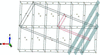Figure 1 Twelve unit cells of sulfur, of type oF, in the intervals u = [−1, 5], v = [0, 1], w = [0, 2]. The unit cell in black is obtained by the transformation a′ = 5a + c; b′ = b; c′ = −a + c, based on the incorrectly simplified Miller and direction indices of the lattice elements (plane, direction) defining the twin lattice, namely (101) and . This unit cell is B centred and has a volume six times larger that the unit cell of the individual crystal, which would suggest a twin index n = 6 × 4/2 = 12, but actually contains lattice nodes also at, 0, 0 and 0, 0,. The real unit cell of the twin lattice is shown in red and is obtained by the transformation a′ = 5a/2 + c/2; b′= b; c′ = −a/2 + c/2, based on the correctly expressed Miller and direction indices of the lattice elements defining the twin lattice, namely (202) and. This unit cell is I centred and is only 1.5 times bigger than the unit cell of the individual crystal, giving the correct twin index n = 1.5 × 4/2 = 3 (figure drawn with VESTA: Momma & Izumi, 2011).

The calculation of the parameters characterizing the twin lattice is usually performed in the primitive unit cell, but in principle this does not ensure avoidance of mistakes coming from approximations of the Miller and direction indices. In fact, as shown in §2, a change of basis from a centred to a primitive unit cell does not correct the mistakes in Miller and direction indices if these are incorrectly expressed in the original cell: it simply makes the error more evident, because in a primitive unit cell Miller indices are indeed relatively prime and direction indices are integers. The condition that the direction indices take integer values in a primitive unit cell is not fulfilled if the corresponding indices are inconsistently expressed in the starting unit cell, in particular when they are unduly forced to fulfil the same restrictions that hold only when the unit cell is primitive.

### 4. Conclusions

The simplification to relatively prime integers which is usually applied to Miller and direction indices is justified only when a primitive unit cell is chosen. In the case of centred cells, however, such a simplification is incorrect, inconsistent with the definition of these indices and a potential source of error. After addressing specifically the case of Miller indices in our previous article (Nespolo, 2015a), we have shown that the same type of problem concerns also crystallographic direction indices: these are actually restricted to rational values. We hope that our analysis will help avoid mistakes and oversights by crystallographic software developers and the end users of such software and draw the attention of crystallography lecturers to the necessity to make students well aware of the consequences of adopting centred cells, which may seem trivial but are actually too often overlooked.

### Footnotes

1A shift of the origin would not have a visible effect in what follows: for this reason we can limit our treatment to the case of a common origin without losing generality.

### Acknowledgements

Critical remarks by three anonymous reviewers are gratefully acknowledged.

### ReferencesJOURNAL OFAPPLIEDCRYSTALLOGRAPHY